# User:Smuratet

Sean Muratet is a GSOC 2012 student from Huntsville, AL, USA. He is currently pursuing a Master of Science degree in Modeling and Simulation at Center for Modeling, Simulation, and Analysis of the University of Alabama in Huntsville. Mr. Muratet graduated from the University of Alabama in Huntsville with a BSE in Chemical Engineering in 2011. He has previously worked as a Graduate Research Assistant at the Center for Modeling, Simulation, and Analysis at UAH.

## GSOC 2012 Goals

1. Complete and test Peng Robinson EOS code currently in the FPROPS 2 branch.
2. Integrate PR code into Ascend
3. Code additional cubic EOS('s) into Ascend, and possible MWBR
4. Continue the work of Richard_Towers on an XML based component file format

## Progress Reports

6 July 2012

To this point, the main effort has been to determine the failure conditions of the current Peng Robinson code. A preliminary investigation dealing strictly with the case of water found that known accurate values of density at temperatures within the 2 phase region return negative pressure values. My first line involved tracing possible unit and conversion errors within the code. This turned out to be a dead end. At this point, I am fairly certain of the accuracy and appropriateness of the units within the PR code, at least as it applies to the saturation and pressure functions. Other function such as enthalpy and entropy have not been fully vetted at this point, but that will come soon. After more debugging, it became apparent that densities outside a certain range returned negative pressure values for a given temperature. This brings up an interesting numerical issue. The PR code takes a density and temperature as arguments to return pressure. This seems to be the tail wagging the dog in a sense. Obviously, at a certain temperature and density, the thermodynamic state of the system is set (Two intensive variables=system defined). However, in the two phase region, more than one density can map to the same pressure. In addition, one density root of the PR equation (the middle value of the three) is not physically significant.

This puts the onus on the user to ensure an appropriate density value is entered, consistent with the PR EOS. This may not be the case in many instances for someone not very familiar with the PR EOS in conjunction with a given fluid. It could be difficult to know a priori how to proceed. I will briefly try to provide examples of common situations which could prove troublesome:

1. Taking the fluid water at 298.15 K and density 997. This is generally known to be a situation where a two phase region is possible. With the PR EOS, this density would return a negative pressure. This could mean one of a few things. Either the fluid is in tension (a physical possibility in general but not here) or we are outside the range of physical significance of the PR EOS. Based on my own investigations, the maximum density must be less than 1/b or in this case approximately 951.752 where the PR EOS exhibits asymptotic behavior. There seems to be an additional asymptote around 2297, beyond which a positive pressure may be found.

2. Under the same temperature conditions, a density of 40 is a root of pressure 1.27 bar. However, this is a pressure root which is not physically significant. The two other roots 12.37 and 850.66 are the physically significant roots. However, without some mechanism to stop it, the user could enter this density and a pressure would be returned which is neither correct nor proper.

3. In general, a cubic will have three roots. For the case of the PR EOS a solution will have three real density roots or one real and two complex roots. If three real roots are found, it seems to indicate a two phase region although there is some disagreement as to whether the phases are in equilibrium as a consequence. If two complex solutions and one real is found, a single phase is indicated with density of the real root. However, a condition arises that is unexpected (to me at least). There is a case when three real roots are returned, but the system should be single phase. For example with carbon dioxide at 300 K and a density of 555, three real roots are returned. one is 555, the other two are -636 and -889.66. Just below at 554, two complex and one real roots (554) are returned. This point at which the transition is made seems to vary with temperature. The pressure from PR EOS is 237 bar (.237 GPa) at 555. This pressure does seem to be well within the range of possibility in terms of a pressure which could be reached. I don't currently know the implications of this behavior, or whether to ignore it or not. It is a situation though which would have to be dealt with in any code to try and find a saturation condition. We then can't simply say if three real roots are returned from a single pressure that the system is two phase and that the highest and lowest roots are the liquid and gas phases, respectively. We could be in the situation just described, and return values of -889.66 and 555 as vapor and liquid densities. Right now I believe it is sufficient to ignore roots which are negative or larger than 1/b. Update: These values were obtained in error on my part. This may not be applicable

Generally speaking, the PR EOS does not describe water very well at all. Still, the same problems might occur for other fluids which are better described. The end user must have some knowledge of equations of state generally to use them. We cannot hope to protect people from the poor results which will come from misuse, while at the same time providing software which is useful. I hope to write code which is a balance between the two principles. At this time, I think that means throwing an error for a density input at or above that which is 1/b. There may be a need to write a more elegant routine, but this should be the beginning.

15 August 2012 The Peng-Robinson EOS has been implemented within FPROPS and is now available from within ascend. The functions of specific volume and temperature implemented include pressure, enthalpy, entropy, internal energy, gibbs energy, helmholtz energy,constant pressure heat capacity, constant volume heat capacity, and fluid sonic velocity. Specifics can be found in the following sections. Many of these functions had been previously coded, but were not working in that a saturation condition could not be found via the implemented code through the PR EOS. I have solved this problem by writing a saturation routine that is specific to cubic eos's, but may well function in other cases. The routine requires an initial guess for vapor phase density provided by the chouaieb correlation. A pressure calculation is made from the PR EOS based on this density, and the liquid phase root is found. The fugacity coefficients of each phase are compared and if not equivalent i.e.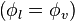$\left(\phi_{l}=\phi_{v}\right)$ then values are successively substituted until equivalence is obtained. Near the critical point, and in some other problem cases, this method alone will not converge. In these cases, Brent's method is used to find the maximum and minimum of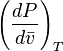$\left(\frac{dP}{d\bar{v}}\right)_{T}$ at the requested temperature. Within these bounds, we can be assured three roots exist. The average of the two pressures (maximum and minimum) is used to continue successive substitution. Using this method, the saturation routine will converge fairly close to the critical point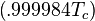$\left(.999984T_{c}\right)$ in tested cases.

20 August 2012 While investigating how to change reference states for the peng robinson correlation values I came upon a fairly big oversight on my part. The enthalpy and entropy functions of the previous code were written with the idea that the current helmholtz fluids files would be used to provide the data the code needs. In the case of the enthalpy function, the ideal portion of enthalpy is calculated using the same function as for the helmholtz correlation. Then a departure function is used to make a correction for non-ideality. In the preparation of the correct run-time data structure in the pengrob code, a call to set the reference state is not being made. Suffice it to say, the enthalpy and entropy functions were being calculated without any regard to reference state. Because I caught this late, I have had to make the changes I thought most prudent. The code overall will be much more useful I think if the fluids from the rpp file can be used in the pengrob code, and not just the fluids containing the helmholtz correlation information. I haven't the time before the stop date to consider how to solve the problem of setting reference states considering that there are possibly two very different sets of fluid information to make the calculations. In addition, the departure function would have to be calculated at the reference state values and then subtracted from the ideal portion. Again, suffice it to say to make the function work properly would require some additional thought and trials. I have therefore hard coded a reference state (NBP) into the enthalpy and entropy functions. To do this we have to find saturated liquid density values at 101325 Pa, as well as temperature. Those values are passed back to the enthalpy and entropy functions so the departure values can be calculated at the desired conditions and at the reference conditions. In testing, I have found that the entropy values from the pengrob code correspond well to the accurate helmholtz correlation values. The enthalpy values returned are generally 5-10% below the helmholtz correlation values. This may be because of a general PR EOS problem with understating liquid densities. A volume translation method implemeted may perhaps help to bring the values more in line. Still, the values seem to follow each other well, regardless of the absolute values. This means that the more important enthalpy difference between states should be relatively close. Generally speaking, absolute enthalpy (and entropy) values are less useful. So, in using this code some points need to be kept in mind: 1. The peng robinson code cannot be used with the helmholtz fluid data values at this point. I hope to fix this in the near future, once I have thought more about how to change reference states for the peng rob code. Use the helmholtz fluid data files for the helmholtz correlations, and the rpp file for the peng robinson code. 2. The values for enthalpy and entropy can only be calculated currently for temperatures between the triple point and the critical temperature using the peng robinson code

15 August 2012

## Overview

The following is some explanation of Peng-Robinson functions implemented within my branch (with some information taken from : The Peng-Robinson EOS is a cubic equation of state in that it contains volume terms to the third power. It is expressed to give pressure in terms of temperature and specific volume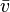${\bar v}$: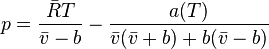$p =\frac{{\bar R} T}{{\bar v}-b}-\frac{a(T)}{{\bar v}({\bar v}+b)+b({\bar v}-b)}$

where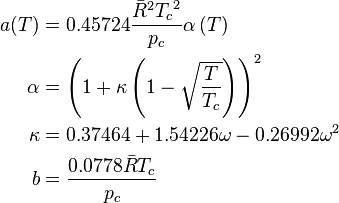\begin{align} a(T) &= 0.45724 \frac{{\bar R}^2{T_c}^2}{p_c} \alpha \left(T \right) \\ \alpha &= \left( 1+\kappa \left( 1-\sqrt{\frac{T}{T_c}} \right) \right)^2 \\ \kappa &= 0.37464+1.54226\omega - 0.26992\omega^2 \\ b &= \frac{0.0778\bar R T_c}{p_c} \end{align}

It is sometimes more convenient to express the equation as a cubic polynomial in terms of compressibility factor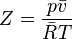$Z=\frac{p {\bar v}}{{\bar R} T}$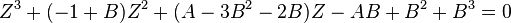$Z^3+(-1+B)Z^2+(A-3B^2-2B)Z-AB+B^2+B^3=0$

in which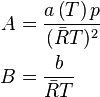\begin{align} A &= \frac{a \left(T \right) p}{({\bar R} T)^2} \\ B &= \frac{b}{{\bar R} T} \end{align}

## Departure Functions

Departure functions represent the departure of the real properties from the ideal properties - i.e the properties of a fluid at zero pressure or infinite molar volume. The departure functions of the Peng-Robinson equation of state are as follows: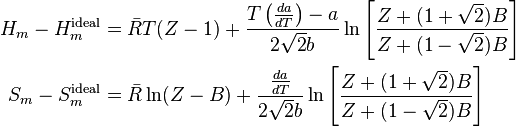\begin{align} H_{m}-H_{m}^{\text{ideal}}&={\bar R} T(Z-1)+\frac{T\left(\frac{da}{dT}\right)-a}{2\sqrt{2}b}\ln\left[\frac{Z+(1+\sqrt{2})B}{Z+(1-\sqrt{2})B}\right] \\ S_{m}-S_{m}^{\text{ideal}}&={\bar R} \ln (Z-B)+\frac{\frac{da}{dT}}{2\sqrt{2}b}\ln\left[\frac{Z+(1+\sqrt{2})B}{Z+(1-\sqrt{2})B}\right] \end{align}

Clearly to evaluate these functions we need to be able to evaluate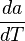$\frac{da}{dT}$ (checked, agrees with Sandler):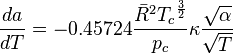$\frac{da}{dT}= -0.45724 \frac{{\bar R}^{2} {T_c}^{\frac{3}{2}} }{p_c} \kappa \frac{\sqrt{\alpha} }{ \sqrt{T}}$

From the above functions, many other properties can be determined by their respective definitions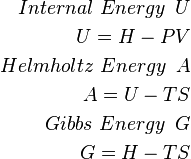\begin{align} Internal~Energy\,~U \\ U=H-PV \\ Helmholtz~Energy\,~A \\ A=U-TS \\ Gibbs~Energy\,~G \\ G=H-TS \\ \end{align}

We can also determine the heat capacities and fluid sonic velocity (speed of sound in a fluid). Currently, the PR EOS in FPROPS uses the rpp.c data file for components by default. This file contains constant pressure heat capacity correlation information. This will provide the ideal portion of the constant pressure heat capacity. The real portion can be determined from the definition: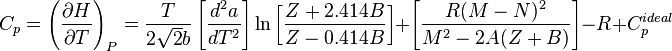$C_{p}=\left(\frac{\partial H}{\partial T}\right)_{P}=\frac{T}{2\sqrt{2}b}\left[\frac{d^{2}a}{dT^{2}}\right]\ln\left[\frac{Z+2.414B}{Z-0.414B}\right]+\left[\frac{R(M-N)^{2}}{M^{2}-2A(Z+B)}\right]-R+{C_{p}^{ideal}}$

where: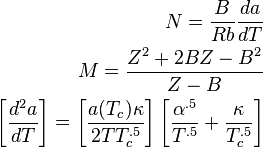\begin{align} N=\frac{B}{Rb}\frac{da}{dT} \\ M=\frac{Z^{2}+2BZ-B^{2}}{Z-B} \\ \left[\frac{d^{2}a}{dT}\right]=\left[\frac{a(T_{c})\kappa}{2TT_{c}^{.5}}\right]\left[\frac{\alpha^{.5}}{T^{.5}}+\frac{\kappa}{T_{c}^{.5}}\right] \\ \end{align}

The ideal part of the constant volume heat capacity can be found from the ideal constant pressure heat capacity as: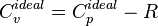$C_{v}^{ideal}=C_{p}^{ideal}-R$

The definition can then be used to find the value: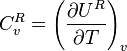${C_{v}^{R}}=\left(\frac{\partial U^{R}}{\partial T}\right)_{v}$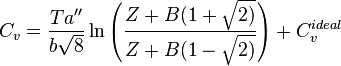$C_{v}=\frac{Ta''}{b\sqrt{8}}\ln\left(\frac{Z+B(1+\sqrt{2)}}{Z+B(1-\sqrt{2)}}\right)+C_{v}^{ideal}$

The speed of sound in a fluid, or the fluid sonic velocity is calculated from: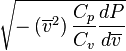$\sqrt{-\left(\overline{v}^{2}\right)\frac{C_{p}}{C_{v}}\frac{dP}{d\overline{v}}}$

## Units

The FPROPS code uses standard SI units.